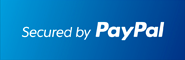﻿ ▷Step by Step Finite Math with the TI89 Calculator ✅ - January, 2022▷Step by Step Finite Math with the TI89 Calculator ✅

Works With TI92 Plus TI89 TI89 Titanium Voyage 200At the end of the PayPal checkout, you will be sent an email containing your key and software.Description

• The most comprehensive Finite Math APP for calculators.
• Step by Step Analysis of..
• Linear Programming
• Marginal Analysis, Demand Analysis
• Markoff Chains
• Simplex Algorithm
• Decision Analysis
• Probabilities
• Combinatorics
• Functions
• Vector and Matrices
• Solve Equations
• Analytic Hierarchy Process
• Logic with Set Theory, Truth Tables
• Relation Analyzer: Find In/Out Degree, Domain , Range, Matrix Mr, Partition, Product sets
• Users have boosted their Finite Math knowledge.
• Ideal for quick review and homework check in Finite Math classes.
• Easy to use. Just plug in the equation and the correct answer shows.

Visit the Classic Site at www.ti89.com/index.htm for more details.

Documentation